Mobile QR Code1. (School of Computer Science and Engineering, Kyungpook National University, Daegu, Korea )

Approximate adder, Approximate computing, Low-cost, Zero truncation, Lower-part OR truncation adder (LOTA)

## 1. Introduction

Continued CMOS technology scaling faces major challenges regarding power and energy consumption, which is one of the critical constraints in designing modern computing systems, such as battery-powered edge devices ($\textit{e.g.,}$ smartphones and smartwatches) . These devices often utilize computationally intensive applications, such as machine learning and multimedia processing . Many of them have built-in error resilience that can tolerate some errors [3-5]. Therefore, approximate computing, which deals with the computation accuracy for power and energy, has received significant interest in recent years [6, 7]. In particular, approximate adders have been studied extensively [8-20].

The error-tolerant adder I (ETAI) consists of an accurate addition part for higher-order input bits and an inaccurate addition part for lower-order counterparts . While the accurate part uses a conventional precise adder, such as ripple carry adder (RCA), the inaccurate part, which performs an approximate addition, uses the modified XOR operation that makes all output bits from a specific bit to the least significant bit (LSB) equal to 1 by checking the most significant bit (MSB) with the LSB of the inaccurate part. The simplified ETA (SETA) optimized the ETAI by simplifying the checking process of the inaccurate part to reduce the power and energy while maintaining accuracy . The lower part OR adder (LOA) is similar to the ETAI in that the adder also has two parts . On the other hand, the difference is that it uses the simple OR operation in the inaccurate part and includes a carry prediction to the accurate part. This improves the overall computation accuracy but degrades the critical path delay. The optimized lower-part OR constant adder (OLOCA) was proposed  to further reduce the power and energy consumption of the LOA by forcing some LSB outputs to 1 regardless of the input values. The carry predicting ETA (CPETA) in  and the enhanced CPETA (ECPETA) in  were developed to improve the accuracy by adding their own proposed low-cost carry prediction methods to the original ETAI architecture, which lacks any carry prediction to the accurate part. The CPETA produces a carry-in signal for the precise adder by an AND operation of the MSB of the lower-part inputs to improve the accuracy. Furthermore, the ECPETA uses both the $\textit{(n-k-1)}$$^{\mathrm{th}} and \textit{(n-k-2)}$$^{\mathrm{th}}$ LSB inputs with an additional OR gate to improve the accuracy further. In , the hybrid error reduction LOA (HERLOA) is presented to enhance the computation accuracy of the LOA by proposing a novel hybrid error reduction scheme for the lower part.

This paper proposes a novel approximate adder using an OR operation and zero truncation to reduce the area, delay, and power and maintain good accuracy. The error rate (ER) of the proposed adder was analyzed mathematically, and its hardware and accuracy performance with various design parameters was investigated. The proposed adder was compared with other adders. The results confirmed the potential competitiveness of the design. Finally, the impact of the error caused by the approximation on digital image processing was analyzed by applying the proposed adder to Gaussian image filtering.

Fig. 1 presents the process and architecture of the proposed approximate adder, termed the lower part OR truncation adder (LOTA). The $\textit{n}$-bit adder splits into a $\textit{k}$-bit accurate part and a $\textit{(n-k)}$-bit inaccurate part. The accurate part exploits a $\textit{k}$-bit precise adder ($\textit{e.g.,}$ RCA) that accurately adds the upper $\textit{k}$-bit inputs. The latter part is composed of OR and zero truncation to add the rest of the inputs approximately. Either of the MSB input bits of the inaccurate part ($\textit{i.e.,}$ $\textit{(n-k-1)}$$^{th} bit) is exploited as the carry (\textit{i.e., C}$$_{in}$) for the precise adder, while the other input bit was used for the output of the corresponding bit position ($\textit{i.e., S}$$_{n-k-1}) as shown in Fig. 1(a). The outputs from \textit{(n-k-1)}$$^{th}$ to $\textit{(l)}$$^{th} bits are produced by OR operation, and the remaining outputs were set to 0 regardless of the inputs. The relatively simpler carry prediction and zero truncation scheme require fewer hardware resources than the existing adders, such as LOA, OLOCA, ETAI, and SETA, which allow a significant decrease in hardware cost. The ER is an important parameter to evaluate the approximate adders, and the ER of the proposed adder was analyzed by considering whether a carry is generated during the addition. Supposing that no carry is generated, an error occurs when \textit{A}$$_{n-k-1}$= 1, or when any $\textit{n-k-1}$ LSB input pairs are both equal to 1, or when any input pairs in the zero truncation part are neither 0. The probability of errors under random inputs in the case is given by

##### (1)
$$P_{\text {case1 }}(n, k, l)=1-\left(\frac{3}{4}\right)^{n-k-l-1}\left(\frac{1}{2}\right)^{2 l+1}$$

In contrast, supposing a carry is generated, an error occurs when the inputs of the OR part are not all 1 because the carry should be propagated from zero truncation part to $\textit{(n-k-1)}$$^{th} bit. Therefore, the probability of errors in the case is given by ##### (2)$$ P_{\text {case } 2}(n, k, l)=1-\left(2^{l}-1\right)\left(\frac{1}{2}\right)^{2 n-2 k-1} $$Considering both cases, the ER of the proposed adder {\textit{ER}}_{LOTA} was derived using the following equation: ##### (3)$$ E R_{\text {LOTA }}(n, k, l)=1-\left(\frac{3}{4}\right)^{n-k-l-1}\left(\frac{1}{2}\right)^{2 l+1}-\left(2^{l}-1\right)\left(\frac{1}{2}\right)^{2 n-2 k-1} $$Fig. 1. (a) Schematic diagram of the operation, (b) general hardware architecture of the proposed approximate adder, lower-part OR truncation adder (LOTA).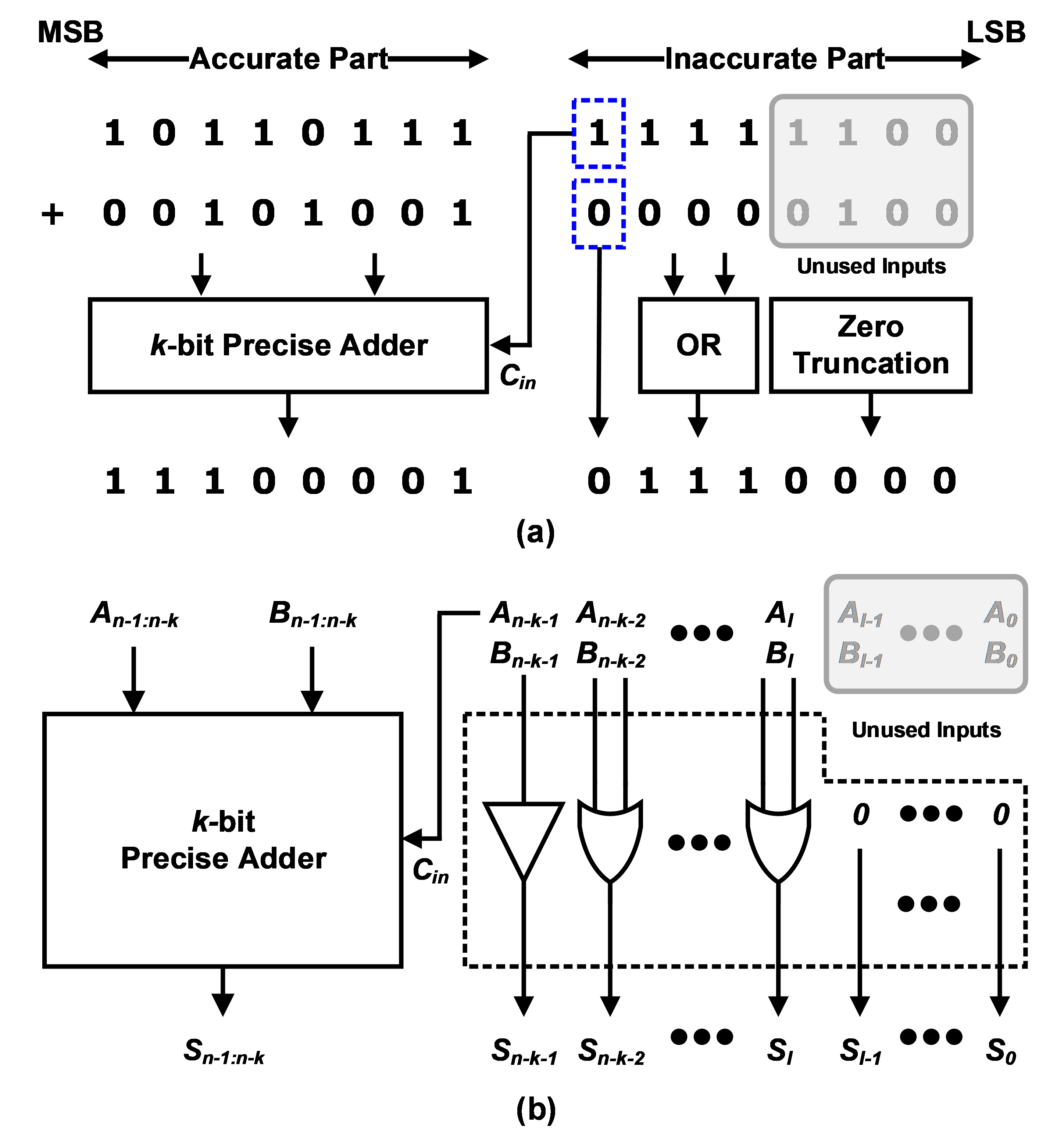Fig. 2. ER of the proposed adder with various values of \textit{l}.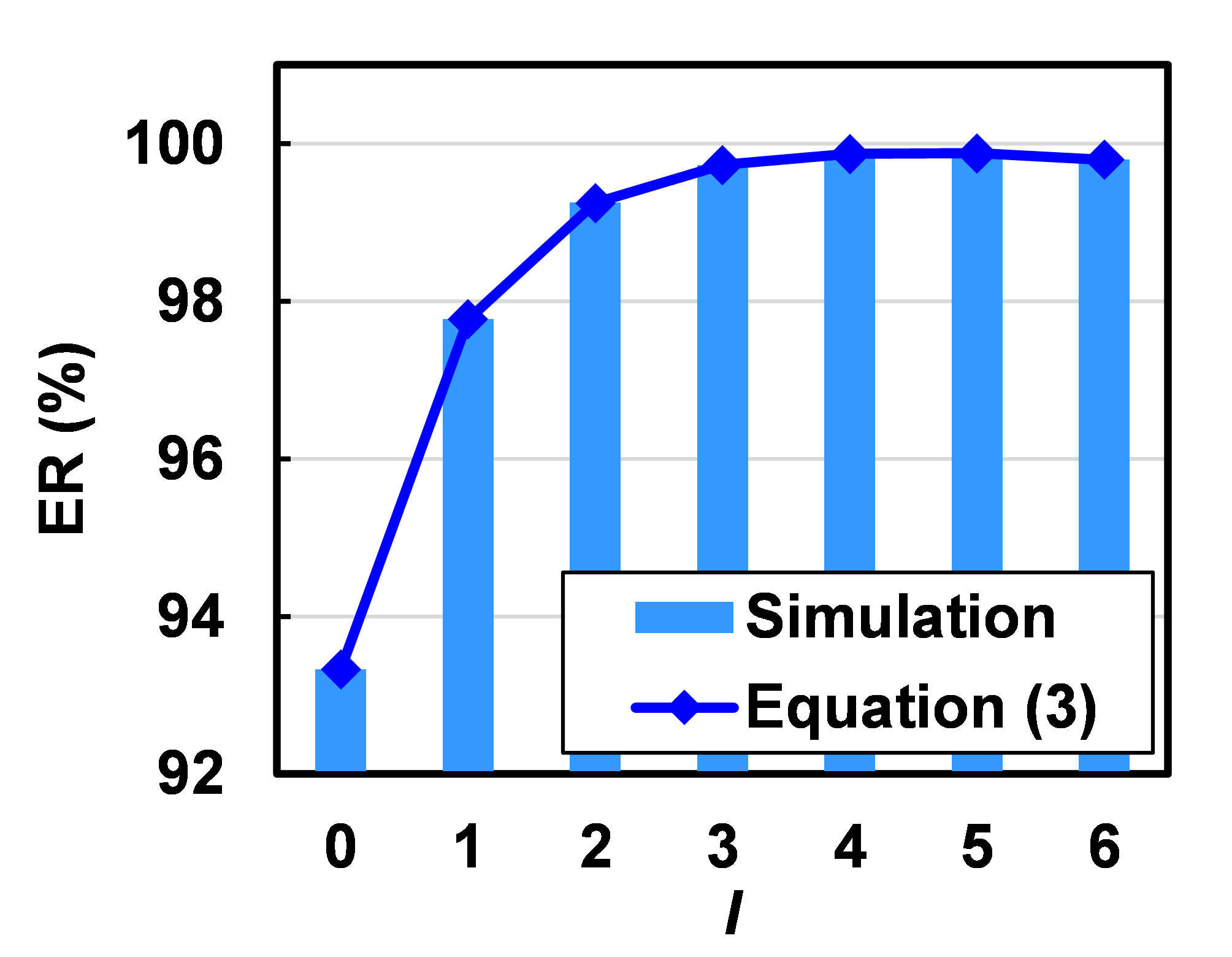Fig. 3. Area-delay-power-NMED product of the proposed adder with various values of \textit{l}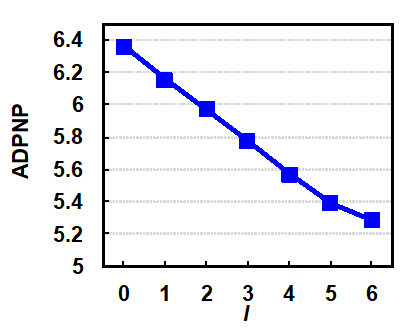Table 1. Performance summary of various adders with \textit{n} = 16 and \textit{k} = 8.  Design Area (µ㎡) Delay (ns) Power (µW) ER (%) NMED (1e-3) RCA 190.4 1.79 58.5 - - LOA 115.8 0.88 33.4 89.99 1.71 OLOCA 102.1 0.88 30.9 99.12 1.77 ETAI 131.2 0.85 33.5 89.99 2.74 SETA 114.2 0.85 30.6 89.99 2.81 LOTA 99.8 0.87 30.7 99.80 1.99 ## 3. Experimental Results The proposed adder was compared with other adders (\textit{i.e.,} RCA, LOA, OLOCA, ETAI, and SETA) regarding both hardware and accuracy. All adders were designed and synthesized using 32-\textit{nm} CMOS technology to test the hardware performance, such as area, delay, and power. The RCA structure was adopted in the precise adder, and the following design parameters were used (\textit{n}=16 and \textit{k}=8). The earlier studies proposed that 7-bit to 9-bit sizes would be suitable for the inaccurate part to obtain a good tradeoff between accuracy and power saving for practical applications (\textit{e.g.,} video and image processing). A 16-bit adder has been adopted widely in these applications [21, 22]. Furthermore, the ER and normalized mean error distance (NMED) were obtained and plotted to evaluate the accuracy of the approximate adders under 10^{7} uniformly distributed random inputs. Fig. 2 presents the ER of the proposed adder with various \textit{l}. From \textit{l}=0 to \textit{l}=5, the ER increased with increasing \textit{l} because the OR part with no carry generation case determines the overall accuracy. On the other hand, when \textit{l}=6, the ER was slightly better than \textit{l}=5 because the case where a carry by zero truncation part is propagated to the accurate part impacts more on the accuracy. Moreover, the line plot obtained from Eq. (1) was introduced in Fig. 2 (a) to see if the derived equation is well matched with the simulation data. The line is in excellent agreement with the simulated ERs at various \textit{l} values. The area-delay-power-NMED product (ADPNP) under various values of \textit{l} was obtained to determine the best tradeoff between the hardware and accuracy performance, as shown in Fig. 3. When the zero truncation part was shortened (\textit{i.e., l} decreases), the proposed adder possessed a better tradeoff and showed the best performance at \textit{l}=6. Therefore, the proposed design with \textit{l}=6 was chosen for comparison with other adders. Table 1 summarizes the performance of the proposed and other adders. As expected, the RCA has the worst hardware performance due to the long carry chain from LSB to MSB with a 1-bit full adder. The LOA has an AND-based carry prediction, whereas the ETAI does not. This makes the LOA more accurate than the ETAI, but it causes a longer delay. In contrast, the OLOCA adjusts some LSB outputs to 1, which requires fewer logic gates than the LOA, so it has a smaller area and less power but worse accuracy. Similarly, the SETA consumes less area and power than the ETAI due to the relatively simpler approximation scheme. The proposed adder reduces the area, delay, and power by 48%, 51%, and 48%, respectively, compared to the RCA. Although the ER of the proposed design approached 100%, its NMED was 27% and 29% better than the ETAI and SETA, respectively, which comparable to those of the LOA and OLOCA. The results showed that the proposed design has the lowest power consumption except for the SETA and the smallest area because this approximation scheme requires fewer logic gates than the others. In addition, directly employing the MSB of the inaccurate part as a carry allows the adder to have a shorter delay than the LOA and OLOCA while maintaining the accuracy. Fig. 4 shows the area-delay product (ADP) versus the power of the approximate adders. The enhanced versions of the ETAI and LOA showed better performance than the original architecture in both ADP and power aspects. The proposed adder was well balanced among the area, delay, and power and showed the best performance in ADP and comparable power to the SETA. The approximate adders were evaluated considering both hardware and accuracy performance by introducing a figure of merit (FOM)  and is defined as ##### (4)$$ F O M=\frac{\text { Energy } \times \text { Delay } \times \text { Area }}{1-N M E D}$$Note that a smaller FOM indicates better tradeoff performance. Fig. 5 shows the FOMs of the proposed and other approximate adders that were normalized against the LOA. The proposed adder had the smallest FOM and the most competitive tradeoff performance. In particular, the proposed adder had a 22.68% lower FOM than the LOA, whose FOM is almost identical to the ETAI. The approximate adders were applied to Gaussian smoothing filtering with a 5$\times \$5 mask to observe the impact of the error of the adders on a digital image processing application. The peak signal-to-noise ratio (PSNR) was calculated to compare the image quality with the adders. The PSNR values were obtained between the Gaussian filtered images with the accurate adder and approximate adders. Note that a higher PSNR value indicates higher similarity. Fig. 6 shows the Gaussian filtered images with an accurate adder, proposed adder, and other approximate adders. The images with the LOA and OLOCA had the same PSNR value of 39.95 dB while those with the ETAI and SETA were the same, which is 26.81 dB. Only the PSNR value of the image with the proposed adder was greater than 40 dB, which is the highest value among the images with the approximate adders. This means that the results of Gaussian filtering with the proposed adder were closest to the filtered result produced by the accurate adder. In addition, the filtered images by the accurate adder and the proposed adder were visually indistinguishable. This proves that the proposed adder is applicable to digital image processing applications because the error caused by an approximation of the proposed adder barely affects the results of Gaussian filtering.

Fig. 4. Area-delay product versus the power of the approximate adders.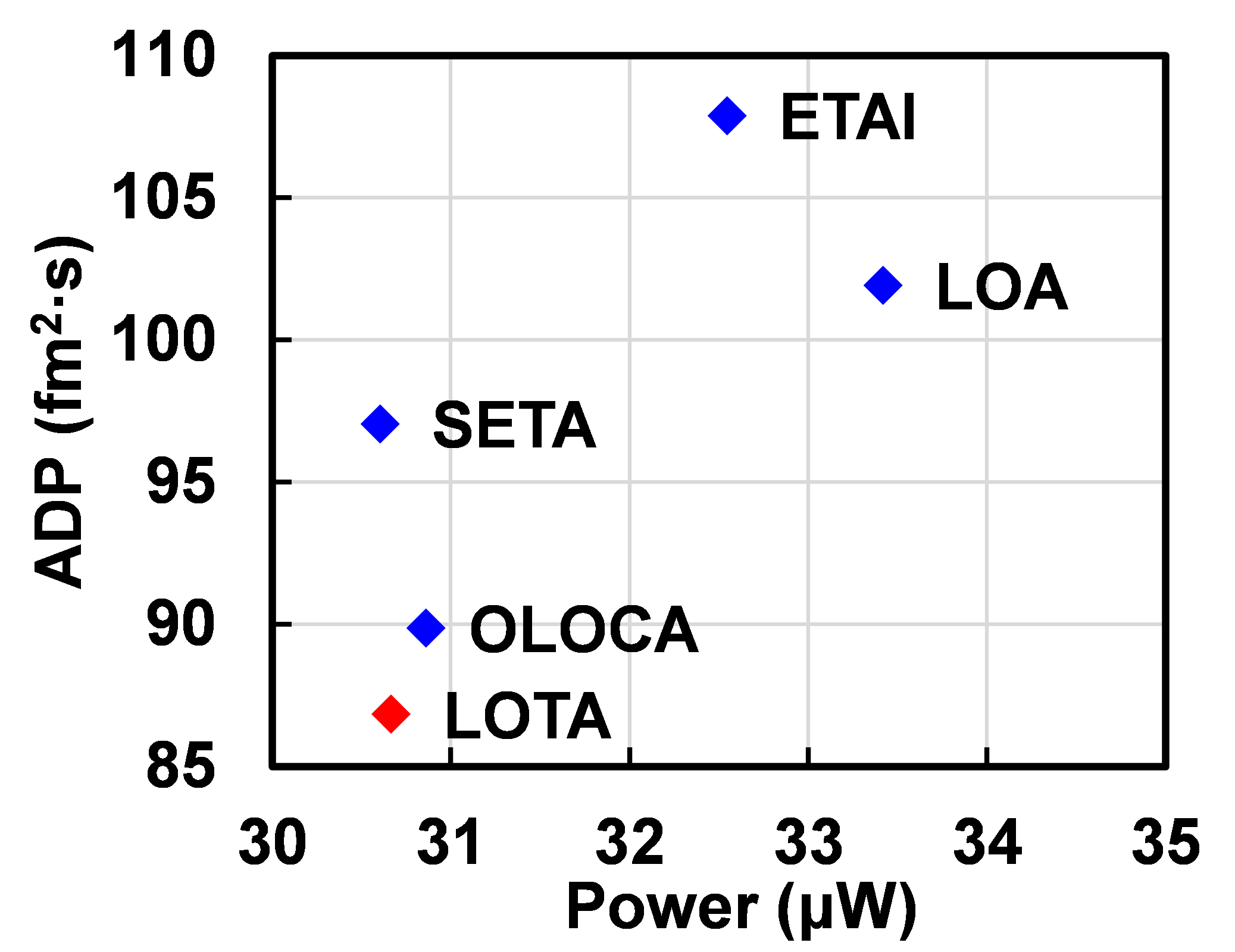Fig. 5. Normalized figure of merit of the approximate adders.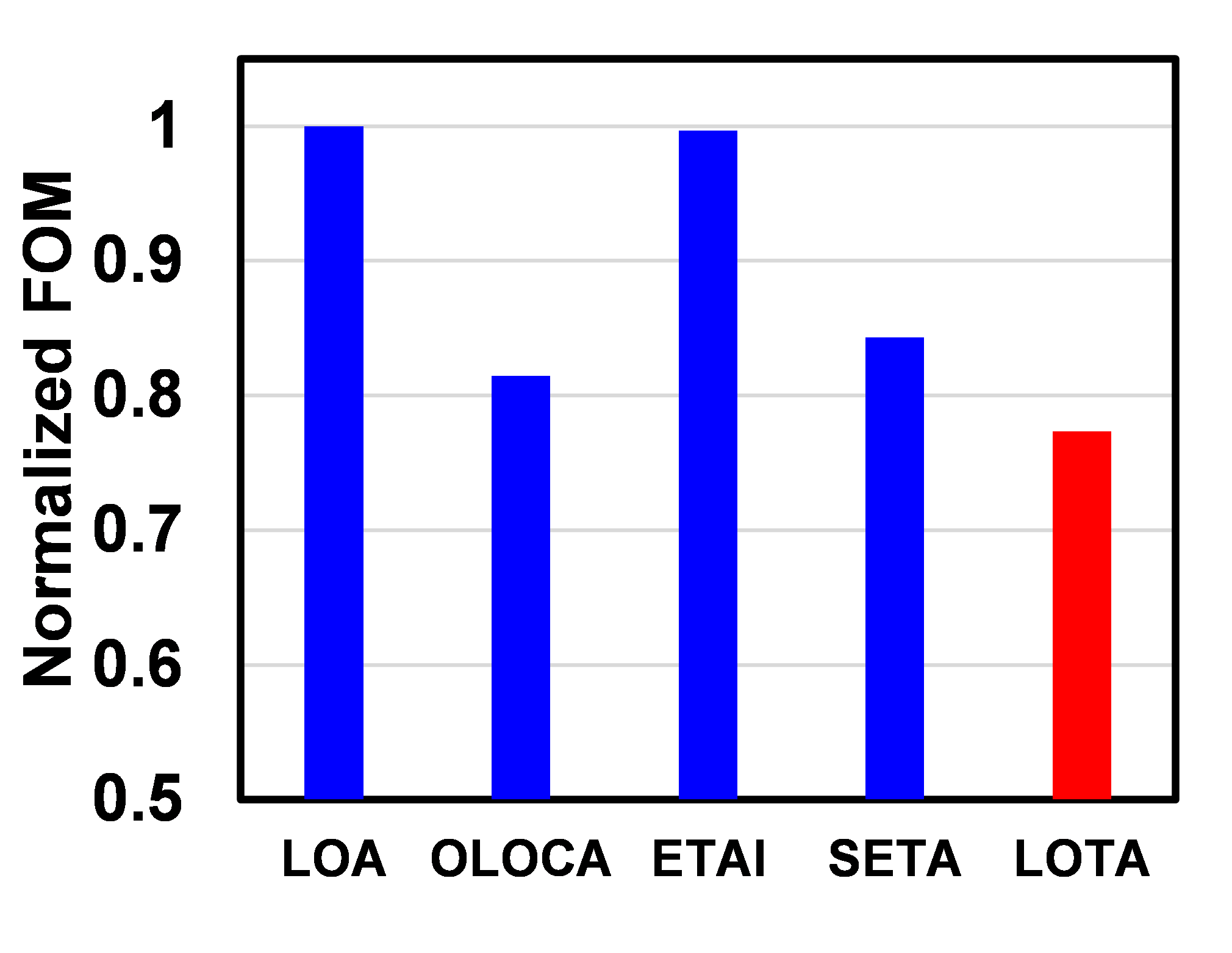Fig. 6. Gaussian filtered images with the accurate adder and approximate adders.## 4. Conclusions

This paper proposed a novel approximate adder that reduces the hardware cost significantly using OR operation and zero truncation. Based on the results, the design has reduced the area, delay, and power by 48%, 51%, and 48%, respectively, compared to the traditional adder RCA. In addition, it showed the best hardware-accuracy tradeoff performance compared to the other approximate adders investigated through the FOM. Gaussian filtering showed that the approximation errors of the proposed design have little impact on the filtered image. The proposed adder has reduced the area and power consumption greatly while providing acceptable accuracy. Therefore, it can be of potential use to enable low-cost approximate computing system design with good energy efficiency.

### ACKNOWLEDGMENTS

This research was supported by Dongil Culture and Scholarship Foundation 2021.

### REFERENCES

1
Jain S., Lin L., Alioto M., 2017, Design-Oriented Energy Models for Wide Voltage Scaling Down to the Minimum Energy Point, IEEE Trans. Circuits. Syst. I, Vol. 64, No. 12, pp. 3115-31252
Wang Q., Li P., Kim Y., 2015, A Parallel Digital VLSI Architecture for Integrated Support Vector Machine Training and Classification, IEEE Trans. Very Large Scale Integr. (VLSI) Syst., Vol. 23, No. 8, pp. 1471-14743
Yang Y., Kim Y., 2020, Approximate Digital Leaky Integrate-and-fire Neurons for Energy Efficient Spiking Neural Networks, IEIE Trans. Smart Process. Comput., Vol. 9, No. 3, pp. 252-2594
Kim Y., Zhang Y., Li P., 2015, A Reconfigurable Digital Neuromorphic Processor with Memristive Synaptic Crossbar for Cognitive Computing, J. Emerg. Technol. Comput. Syst., Vol. 11, No. 4, pp. 38:1-38:255
Wang Q., Kim Y., Li P., Aug. 2014, Architectural Design Exploration for Neuromorphic Processors with Memristive Synapses, IEEE Int. Conf. Nanotechnology, pp. 962-9966
Xu S., Schafer B. C., 2019, Toward Self-Tunable Approximate Computing, IEEE Trans. Very Large Scale Integr. (VLSI) Syst., Vol. 27, No. 4, pp. 778-7897
Raha A., et al. , 2017, Quality Configurable Approximate DRAM, IEEE Trans. Comput., Vol. 66, No. 7, pp. 1172-11878
Zhu N., et al. , 2010, Design of Low-Power High-Speed Truncation-Error-Tolerant Adder and its Application in Digital Signal Processing, IEEE Trans. Very Large Scale. Integr. (VLSI) Syst., Vol. 18, No. 8, pp. 1225-12299
Lee J., et al. , 2020, Approximate Adder Design with Simplified Lower-Part Approximation, IEICE Electron. Express, Vol. 17, No. 15, pp. 1-310
Mahdiani H. R., et al. , 2010, Bio-Inspired Imprecise Computational Blocks for Efficient VLSI Implementation of Soft-Computing Applications, IEEE Trans. Circuits. Syst. I, Vol. 57, No. 4, pp. 850-86211
Dalloo A., et al. , 2018, Systematic design of an approximate adder: the optimized lower part constant-OR adder, IEEE Trans. Very Large Scale. Integr. (VLSI) Syst., Vol. 26, No. 8, pp. 1595-159912
Kim Y., 2019, An Accuracy Enhanced Error Tolerant Adder with Carry Prediction for Approximate Computing, IEIE Trans. Smart Process. Comput., Vol. 8, No. 4, pp. 324-33013
Kim Y., 2019, A Novel Approximate Adder with Enhanced Low-cost Carry Prediction for Error Tolerant Computing, IEIE Trans. Smart Process. Comput., Vol. 8, No. 6, pp. 506-51014
Seo H., Yang Y. S., Kim Y., 2020, Design and Analysis of Approximate Adder with Hybrid Error Reduction, Electronics, Vol. 9, No. 3, pp. 471:1-471:1315
Akbari O., et al. , 2018, RAP-CLA: A Reconfigurable Approximate Carry Look-Ahead Adder, IEEE Trans. Circuits. Syst. II: Exp. Briefs, Vol. 65, No. 8, pp. 1089-109316
Kim Y., Zhang Y., Li P., Nov. 2013, An Energy Efficient Approximate Adder with Carry Skip for Error Resilient Neuromorphic VLSI Systems, in IEEE/ACM Int. Conf. Comput.-Aided Design, pp. 130-13717
Kim Y., Zhang Y., Li P., 2015, Energy Efficient Approximate Arithmetic for Error Resilient Neuromorphic Computing, IEEE Trans. Very Large Scale. Integr. (VLSI) Syst., Vol. 23, No. 11, pp. 2733-273718
Lee J., Seo H., Kim Y., Oct. 2020, Design of a Low-Cost Approximate Adder with a Zero Truncation, Int. SoC Design Conf., pp. 69-7019
Seo H., Yang Y. S., Kim Y., Oct 2020, An Energy-Efficient Imprecise Adder with a Lower-part Constant Approximation, Int. SoC Design Conf., pp. 143-14420
Seo H., Kim Y., Nov. 2021, A New Approximate Adder with Duplicate-Constant Scheme for Energy Efficient Applications, IEEE Int. Conf. Consumer Electronics-Asia, pp. 1-221
Gupta V., et al. , 2013, Low-Power Digital Signal Processing Using Approximate Adders, IEEE Trans. Comput.-Aided Design Integr. Circuits Syst., Vol. 32, No. 1, pp. 124-13722
Raha A., Jayakumar H., Raghunathan V., 2016, Input-Based Dynamic Reconfiguration of Approximate Arithmetic Units for Video Encoding, IEEE Trans. Very Large Scale Integr. (VLSI) Syst., Vol. 24, No. 3, pp. 846-857## Author

Hyoju Seo received the B.S. degree from the School of Computer Science and Engineering, Kyungpook National University, Daegu, Republic of Korea in 2020, where she is pursuing an M.S. degree. Her research interests include artificial intelligence (AI), computer architecture, approximate computing, and image processing.

Jungwon Lee is currently pursuing the integrated B.S. and M.S. degrees in the School of Computer Science and Engineering at Kyungpook National University, Daegu, Republic of Korea. Her research interests include deep learning, new computing systems, and approximate arithmetic

Donghui Lee received his B.S. degree in the Department of Electric and Electronic Engineering from Halla University, Wonju, Republic of Korea in 2020. He is pursuing an M.S. degree in the School of Computer Science and Engineering at Kyungpook National University, Daegu, Republic of Korea. His research interests include artificial intelligence (AI) accelerator and approximate computing.

Beomjun Kim received his B.S. degree from the School of Computer Science and Engineering from Kyungpook National University, Daegu, Republic of Korea in 2021, where he is pursuing an M.S. degree. His research interests include computer architecture, non-volatile memory, data compression, and heterogeneous memory system.

Yongtae Kim received the B.S. and M.S. degrees in electrical engineering from the Korea University, Seoul, Republic of Korea, in 2007 and 2009, respectively, and the Ph.D. degree from the Department of Electrical and Computer Engineering from the Texas A&M University, College Station, TX, in 2013. From 2013 to 2018, he was a software engineer with Intel Corporation, Santa Clara, CA. Since 2018, he has been with the School of Computer Science and Engineering at Kyungpook National University, Daegu, Republic of Korea, where he is currently an assistant professor. His research interests are energy-efficient integrated circuits and systems, particularly neuromorphic computing and approximate computing, and new memory devices and architectures.